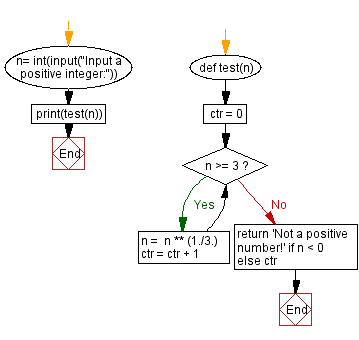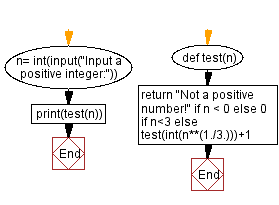﻿ Python - Iterated Cube Root

# Python Exercises: Iterated Cube Root

## Python Basic - 1: Exercise-150 with Solution

Write a Python program that takes a positive integer and calculates the cube root of the number until the number is less than three. Count the number of steps to complete the task.

Sample Data:

(3) -> 1
(39) -> 2
(10000) -> 2

Sample Solution-1:

Python Code:

``````def test(n):
ctr = 0
while n >= 3:
n =  n ** (1./3.)
ctr = ctr + 1
return 'Not a positive number!' if n < 0 else ctr

n= int(input("Input a positive integer:"))
print(test(n))
``````

Sample Output:

```Input a positive integer: 3
1
Input a positive integer: 39
2
Input a positive integer: 10000
2
Input a positive integer: -4
Not a positive number!
```

Flowchart:Sample Solution-2:

Python Code:

``````def test(n):
return "Not a positive number!" if n < 0 else 0 if n<3 else test(int(n**(1./3.)))+1
n= int(input("Input a positive integer:"))
print(test(n))
``````

Sample Output:

```Input a positive integer: 14
1
Input a positive integer: -5
Not a positive number!
```

Flowchart:Python Code Editor:

Have another way to solve this solution? Contribute your code (and comments) through Disqus.

What is the difficulty level of this exercise?

Test your Programming skills with w3resource's quiz.

﻿